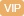| APP | 校园号 | 客服 客服热线：400-863-9889

400-863-98892000-619=7.2÷4=
 3 4
+
 2 3
=
 8 9
×
 1 4
=
0.3×0.04=
 8 9
÷
 4 9
=
1÷0.25=4-
 5 7
=
60÷60%=0.53=23×498≈803-207≈
 3 5
×
 2 3
÷
 3 5
×
 2 3
=
2.25-0.45-1.55=
【考点】整数的加法和减法数的估算分数的加法和减法分数的四则混合运算小数除法【专题】计算题．
【分析】根据数分数小数加减乘的计算方法估算的方法进行计；225-045-15，按照减法的性质算．
【解答】解：
200-61=13817.2÷4=8
 3 4
+
 2 3
=
 17 12
 8 9
×
 1 4
=
 2 9
0.3.0=0.012
 8 9
÷
 4 9
=2
1÷025=44-
 5 7
=3
 2 7
060%=100053=012523×98≈1000803207≈60
 3 5
×
 2 3
÷
 3 5
×
 2 3
=
 4 9
.25-.4-1.55=0.5
【点评】口算时，注意运算号和数据然后再一步算．

0/0